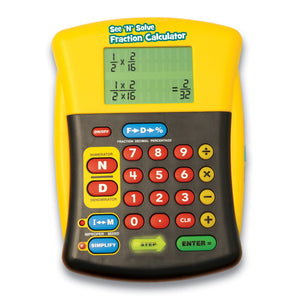# See & Solve Fraction Calculator 顯示步驟計數機 - 份數

Regular price
Sold out
Sale price
\$295.00
Quantity must be 1 or more

The See ‘n’ Solve Fraction Calculator is the only user-friendly calculator on the market that shows students how to solve a fraction problem, step by step. The easy-to-use format reinforces introductory fraction skills without the complications of other scientific calculators—making it perfect for school and home. Students can add, subtract, multiply, and divide fractions easily. Press the special Numerator and Denominator keys to input a fraction problem. The large 2-line screen displays the problem (with common denominator), and lighted keys guide students through the steps to solve it. Special keys instantly simplify fractions or convert fractions to decimals or percentages. A terrific way for students to see and understand how to solve any fraction problem. Requires 2 AA batteries (not included).

．Finish your homework in a fraction of the time.
．The easiest-to-use fraction calculator out there!
．Reinforces introductory fraction skills.
．Big LCD screen shows step-by-step solutions.
．Perfect for home and school.

Senior： 份 數 運 算 計 數 機
★ 可顯示標準直式步驟及答案
★ 份數運算計算機備有一本12頁使用指南
★ 輕巧便攜, 大約呎吋: 闊5'' x 高6''
★ 物料: 耐用塑膠外款
★ 須配合電池使用
★ 特大電子屏幕顯示
★ 6歲或以上使用 (可按程度提早或推遲使用)
★ 100%原裝美國入口